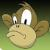## 一些无聊的效率问题

1）计算1到1亿的和，java费时120毫秒，php费时23秒，两者相差近200倍。

2）在java中，将整数i转换成字符串，可以i+""也可以Integer.toString(i)，前者耗时是后者的2.5倍。

3）在java中，遍历字符串中各个字符并进行比较，可以将字符串拆解成字符数组进行遍历，也可以用String.charAt()方法进行遍历，遍历时后者耗时是前者的1.5倍。但是前者需将字符串拆解成字符数组也需要时间，加上这部分时间，前者反而是后者的1.7倍。

0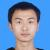0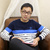```public class Sum {
public static void main(String[] args) {
int sum = 0;
for (int i = 1; i <= 100000000; i++) {
for (int n = i; n > 0; n /= 10) {
if (n % 10 == 1) {
sum++;
}
}
}
System.out.println(sum);
}
}```

0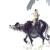#### 引用来自“redraiment”的答案

```public class Sum {
public static void main(String[] args) {
int sum = 0;
for (int i = 1; i <= 100000000; i++) {
for (int n = i; n > 0; n /= 10) {
if (n % 10 == 1) {
sum++;
}
}
}
System.out.println(sum);
}
}```

```		int total = 0;
for(int i = 1; i <= 100000000; i++){
String number = Integer.toString(i);
int length = number.length();
for (int j = 0; j < length; j++) {
if (number.charAt(j) == '1') {
total++;
}
}
}```

01)先除(除数为变数)后求模，效率最高，为8.611秒
2)直接操作字符串，其次，为9.861秒
3)先除(除数为定数)后求模，最小最低，为11.544秒。

0#### 引用来自“唐明星”的答案

1)先除(除数为变数)后求模，效率最高，为8.611秒
2)直接操作字符串，其次，为9.861秒
3)先除(除数为定数)后求模，最小最低，为11.544秒。

80000001，费时:11441
80000001，费时:4536
80000001，费时:10942

0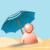1、php是解释性语言，而Java说是说是解释性的，但其实它是半编译型的，Java编译器会把源码编译成一种介于机器码和源码的class文件，这样的处理让Java很多特性接近于c语言，有人研究甚至有些特性超过c

2、对于web工程来说，应该是IO密集型而不是CPU密集型的，如果你的工程是CPU密集型的话，你就应该好好审查一下你的工程设计在那里有问题了。@唐明星 : 另外我举web工程的意思是指一个实际的应用。什么算法，效率都是要根据实际应用来的，没有实际应用价值的东西讨论没有什么意义。1.php也是先编译成中间代码，然后解释执行的，只是java的叫字节码，php的叫操作码，名字不同，实质一样。你所看到的是假象，只知java有bytecode，而不知道php有opcode，前者是面向程序员，后者php有意隐藏了而已（php代码缓存器，比如APC，就是缓存的opcode，防止重复解释）。 2.不是web工程，一个普通算法而已。况且我说过了，这些细节不影响程序，只是作为研究而言罢了。
0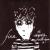```    public static int computeBit4() {
int total = 0;
final int BIT_MAP_SIZE = 10000;
int[] bitMap = new int[BIT_MAP_SIZE];
for (int i = 1; i <= 100000000; i++) {
if (i < BIT_MAP_SIZE) {
int count = 0;
for (int j = 1; j <= i; j *= 10) {
if (i / j % 10 == 1) {
count++;
}
}
bitMap[i] = count;
total += count;
} else {
int num = i;
while (num > 0) {
total += bitMap[num % BIT_MAP_SIZE];
num /= BIT_MAP_SIZE;
}
}
}
}

public static int computeBit5() {
int total = 0;
final int BIT_MAP_SIZE = 10000;
int[] bitMap = new int[BIT_MAP_SIZE];
for (int i = 1; i <= 100000000; i++) {
if (i < BIT_MAP_SIZE) {
int count = 0;
for (int j = 1; j <= i; j *= 10) {
if (i / j % 10 == 1) {
count++;
}
}
bitMap[i] = count;
total += count;
} else if (i != 100000000) {
total += bitMap[i % BIT_MAP_SIZE] + bitMap[i / BIT_MAP_SIZE];
} else {
total += 1;
}
}
}```

0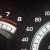0#### 引用来自“xyz555”的答案

1、php是解释性语言，而Java说是说是解释性的，但其实它是半编译型的，Java编译器会把源码编译成一种介于机器码和源码的class文件，这样的处理让Java很多特性接近于c语言，有人研究甚至有些特性超过c

2、对于web工程来说，应该是IO密集型而不是CPU密集型的，如果你的工程是CPU密集型的话，你就应该好好审查一下你的工程设计在那里有问题了。

0